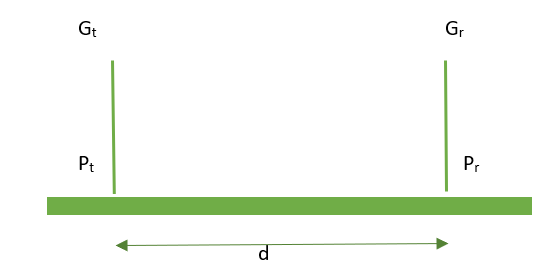GFG App
Open AppBrowser
Continue

# Friis Equation in Wireless Communication

The Friis Equation is a mathematical formula used in the field of wireless communication engineering to calculate the power received at a receiver antenna from a transmitter antenna. The equation provides an estimate of the power received at the receiver antenna by considering the losses that occur during the propagation of the electromagnetic waves in free space. It is a widely used formula in wireless communication that relates the power transmitted from a transmitting antenna to the power received at a receiving antenna, taking into account the distance between them and other factors that affect the signal propagation. The equation is named after Danish engineer Harald T. Friis, who developed it in the 1940s.

The Friis equation is expressed as follows:

`Pr = Pt * Gt * Gr * (lambda / 4 * pi * d)^2`

where:

• Pr is the power received at the receiving antenna in watts
• Pt is the power transmitted from the transmitting antenna in watts
• Gt is the gain of the transmitting antenna
• Gr is the gain of the receiving antenna
• lambda is the wavelength of the signal in meters
• d is the distance between the transmitting and receiving antennas in meters
• pi is the mathematical constant pi

The Friis equation is used in various applications of wireless communication, such as radio communication, satellite communication, and wireless networking. It is used to estimate the strength of the received signal and to optimize the antenna configuration for maximum signal strength. The equation assumes free space propagation and does not take into account signal attenuation due to obstacles, reflection, and diffraction, which can have a significant impact on the actual received signal strength.

### Derivation of the Friis Equation:

The Friis Equation is based on the inverse square law, which states that the power received at a given point in space is proportional to the inverse square of the distance between the transmitter and receiver. The equation can be derived as follows:Power transmitted from the transmitter antenna: The power transmitted from the transmitter antenna is given by the equation,

``

Where Pt is the power transmitted from the transmitter antenna, Gt is the gain of the transmitter antenna, λ is the wavelength of the electromagnetic wave, and d is the distance between the transmitter and receiver.Where Pr is the power received at the receiver antenna, Gr is the gain of the receiver antenna, and d is the distance between the transmitter and receiver.

Free Space Loss: The loss in power that occurs due to the propagation of the electromagnetic waves in free space is given by the equation,where Lfs is the free space loss and d is the distance between the transmitter and receiver.

By combining the above equations, we can derive the Friis Equation: Pr = PtGrGr / (λ^2Lfs) = PtGrGr / (4πd)^2

Here,

```Pt = power applied to the transmitting antenna
Pr =  power received at the receiving antenna
Gr = Gain of the receiving antenna.
Gt =  Gain of the transmitting antenna.```

The Friis Equation provides a useful estimate of the power received at the receiver antenna in free space and is widely used in the design and analysis of wireless communication systems.

In conclusion, the Friis Equation is a fundamental tool in the field of wireless communication engineering, providing a way to estimate the power received at a receiver antenna from a transmitter antenna in free space. Its derivation is based on the inverse square law, which states that the power received is proportional to the inverse square of the distance between the transmitter and receiver.

My Personal Notes arrow_drop_up